# 2D drawing – plots and graphs in Matlab

 Matlab 2D drawing or plotting functions and tools direct their output to a window that is separate from the command window (referred to as a figure). By default, Matlab uses line styles and colors to distinguish the data sets plotted in the graph. You can change the appearance of the graphic components or add annotations to the graph to explain your data for presentation.

Below you can find examples of some 2D drawing functions. Many of them take optional arguments. These are just some ideas to give you basic understanding or how to use these built-in functions and what to expect from them. For more information, see the online Matlab help (type ‘help function’ on your command window or see the Help menu).  Some of these examples have a full article on those functions.

 fplot fplot('x.*cos(5*x)', [0 4*pi])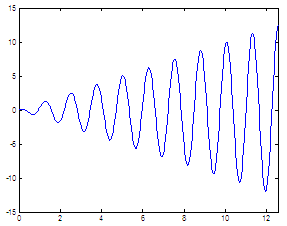semilogx x=0 : .01 : 2*pi; semilogy(x,exp(2*x))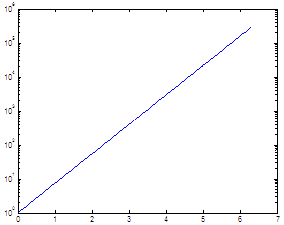t = 0 : .01 : pi; x = exp(2*t); y = 50+exp(t); loglog(x,y) grid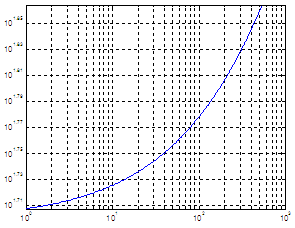t=0 : .01 : 2*pi; r=sqrt(abs(sin(3*t))); polar(t,r)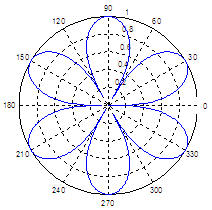bar x = 0 : 10; y = 2*x+3; bar(x,y)plotyy x = 1:.1:10; y1=exp(-2*x).*cos(x); y2=exp(2*x); H =plotyy(x,y1,x,y2); h1=get(H(1),'ylabel'); h2=get(H(2),'ylabel'); set(h1,'string','fun y1'); set(h2,'string','fun y2');x = -2*pi:.01:2*pi; y = sin(x)./x; area(x,y)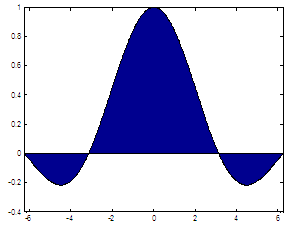dat = [5 5 20 30 40]; pie(dat)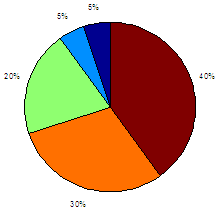y = 20*rand(1,10); hist(y)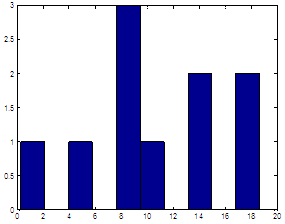t = -3*pi : 3*pi; y = cos(t)-2*sin(t); stem(t,y)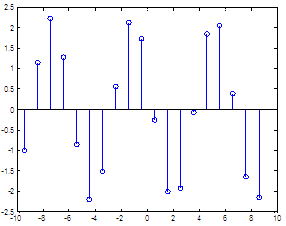stairs x = 1 : 10; y = 2*x; stairs(x,y)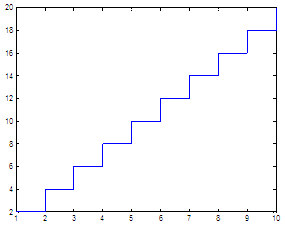t = 0 : pi/6 : pi; x = cos(t); y = i*sin(t); z = x + y; compass(z)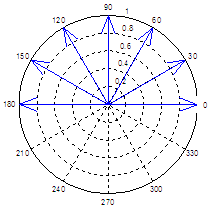X = -3 : .1 : 3; [x,y]=meshgrid(X,X); z = -x^2 + 2*x.*y + y^2; pl=contour(x,y,z); clabel(pl)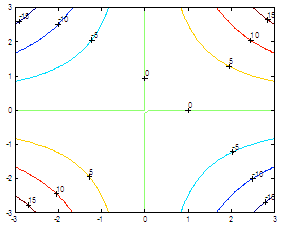### Video - Create 2D Images in Matlab

From '2D drawing' to home

From '2D drawing' to Plot Menu